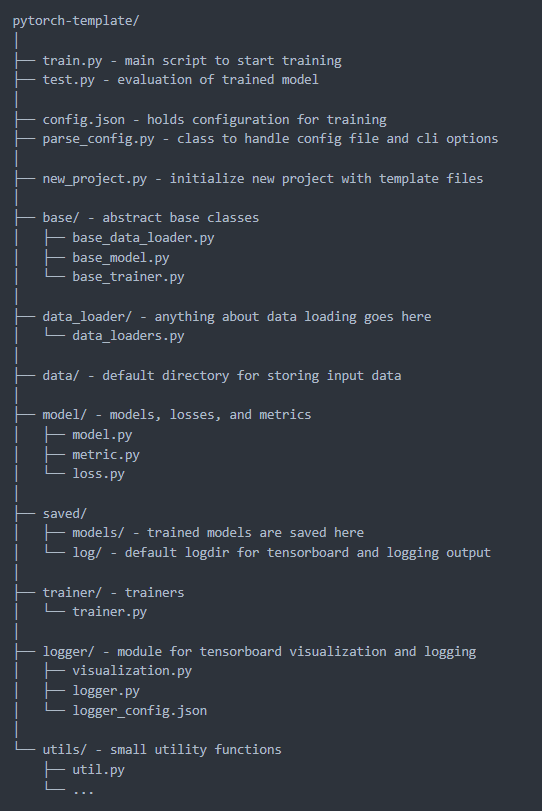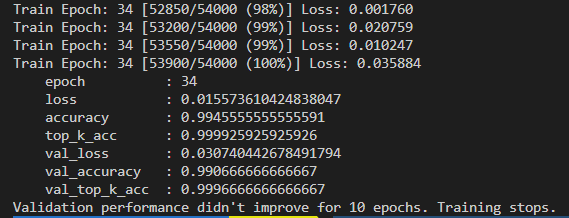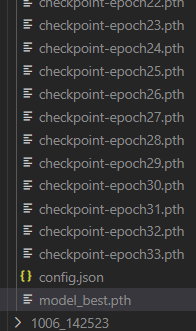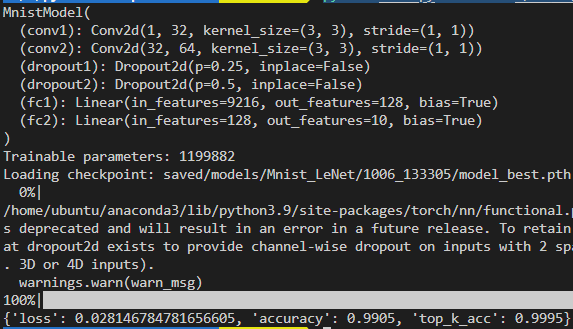# [GDSC-ML] Apply PyTorch template to Mnist classification

bona-park·2022년 10월 6일
0The second GDSC-ML session was to convert Jupyter Notebook of MNIST CNN model into Python scripts.

Like most people, I was used to do ML projects through Jupyter notebook. It had a big advangtage that I can validate and check the code easily by just typing (Shift + Enter).

But there are some fallbacks of Jupyter Notbooks in data science projects

• Unorganized
• hard to keep track of what I write
• Not ideal for reproducibility
• if there's a slight data change, hard to identify a source error
• Not ideal for production
• hard to run Jupyter Notebook while using other tools

This time I used @victoresque 's Pytorch-Template.
It provides a clear folder structure suitable for many Deep Learning Projects.### previous mnist_examle.ipynb outline

• set device
• hyperparmeter setting
• see dataset(we don't need it)
• use torch dataloader to divide dataset into mini-batch
• define 2 Dataloaders: train_loader test_loader
• define Basic CNN class
• create model instance with preconifigured class
• define optimizer -> mutate in 'config.json'
• choose Loss function
• train the model
• update gradient in each training steps
• evaluate the model

Because both projects are dealing with MNIST, there weren't that many things to change from the template.

We only need to change some parameters in config.json file and change the model structure in model.py

### New Python Scripts using a template

#### config.json

{
"name": "Mnist_LeNet",
"n_gpu": 1,

"arch": {
"type": "MnistModel",
"args": {}
},
"args": {
"data_dir": "data/",
"batch_size": 50,
"shuffle": true,
"validation_split": 0.1,
"num_workers": 2
}
},
"optimizer": {
"args": {
"lr": 0.0001,
"weight_decay": 0,
}
},
"loss": "nll_loss",
"metrics": ["accuracy", "top_k_acc"],
"lr_scheduler": {
"type": "StepLR",
"args": {
"step_size": 50,
"gamma": 0.1
}
},
"trainer": {
"epochs": 100,

"save_dir": "saved/",
"save_period": 1,
"verbosity": 2,

"monitor": "min val_loss",
"early_stop": 10,

"tensorboard": true
}
}


#### model.py

class MnistModel(BaseModel):
def __init__(self): ..
def forward(self, x): ..

#### BaseModel.py

class BaseModel(nn.Module):
"""
Base class for all models
"""
@abstractmethod
def forward(self, *inputs):
"""
Forward pass logic
:return: Model output
"""
raise NotImplementedError

#### train.py

# setup data_loader instances with following conditions in config.json

# build model architecture, then print to console
model = config.init_obj("arch", module_arch)
logger.info(model)

# prepare for (multi-device) GPU training
device, device_ids = prepare_device(config["n_gpu"])
model = model.to(device)
if len(device_ids) > 1:
model = torch.nn.DataParallel(model, device_ids=device_ids)

# get function handles of loss and metrics
criterion = getattr(module_loss, config["loss"])
metrics = [getattr(module_metric, met) for met in config["metrics"]]

# build optimizer, learning rate scheduler. delete every lines containing lr_scheduler for disabling scheduler
trainable_params = filter(lambda p: p.requires_grad, model.parameters())
optimizer = config.init_obj("optimizer", torch.optim, trainable_params)
lr_scheduler = config.init_obj("lr_scheduler", torch.optim.lr_scheduler, optimizer)

trainer = Trainer(
model,
criterion,
metrics,
optimizer,
config=config,
device=device,
lr_scheduler=lr_scheduler,
)
trainer.train()

Copies parameters and buffers from state_dict into this module and its descendants. If strict is True, then the keys of state_dict must exactly match the keys returned by this module’s state_dict() function.path: ./saved/models/Mnist_LeNet/1006_133305/model_best.pth

#### test.py

    data_loader = getattr(module_data, config["data_loader"]["type"])(
batch_size=50,  #
shuffle=True,  #
validation_split=0.0,
training=False,
num_workers=2,
)

# build model architecture
model = config.init_obj("arch", module_arch)
logger.info(model)

# get function handles of loss and metrics
loss_fn = getattr(module_loss, config["loss"])
metric_fns = [getattr(module_metric, met) for met in config["metrics"]]

state_dict = checkpoint["state_dict"]
if config["n_gpu"] > 1:
model = torch.nn.DataParallel(model)

# prepare model for testing
device = torch.device("cuda" if torch.cuda.is_available() else "cpu")
model = model.to(device)
model.eval()

total_loss = 0.0
total_metrics = torch.zeros(len(metric_fns))

for i, (data, target) in enumerate(tqdm(data_loader)):
data, target = data.to(device), target.to(device)
output = model(data)

#
# save sample images, or do something with output here
#

# computing loss, metrics on test set
loss = loss_fn(output, target)
batch_size = data.shape
total_loss += loss.item() * batch_size
for i, metric in enumerate(metric_fns):
total_metrics[i] += metric(output, target) * batch_size

logger.info(log)
Running Command: python test.py --resume ./saved/models/Mnist_LeNet/1006_133305/model_best.pth